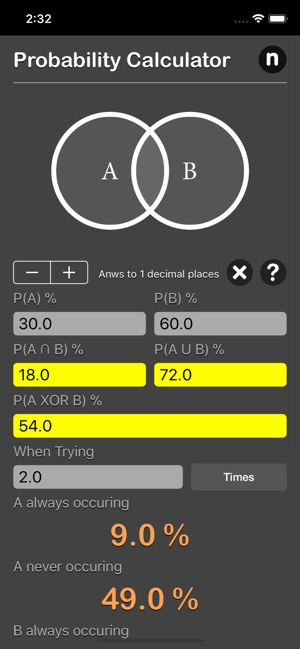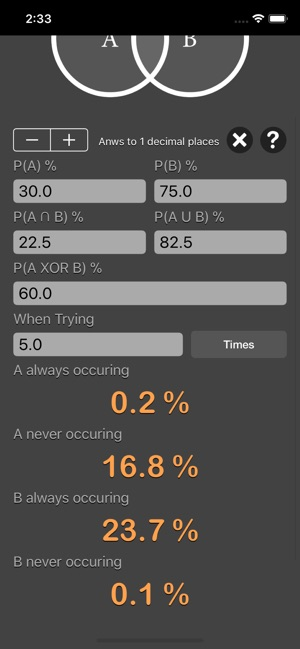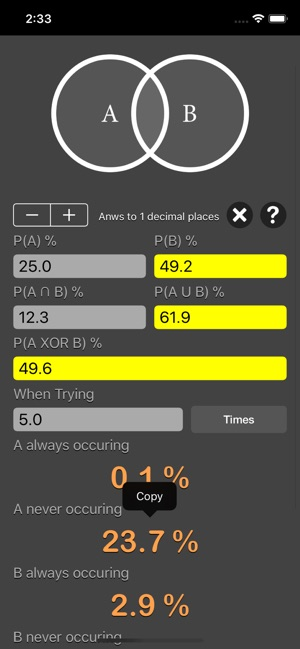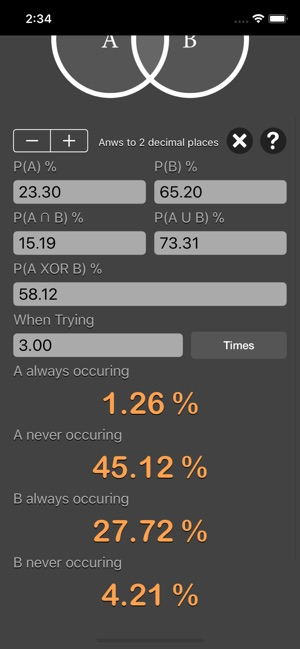## Screenshots

••••••## Description

Probability Calculator are math calculator to find the chance that the given event will occur.

Find the relationships of two separate events. For example, if the the chances of A happening is 40%, and 50% for B, what are the chances of both happening, or one of them occur, or neither, and so on.

Features:
- Instant calculation
- Result are copy able to other app
- Formula are include as reference
- Support up to 16 decimal place

Probability is the measure of the likelihood that an event will occur. Probability is quantified as a number between 0 and 1, where, loosely speaking, 0 indicates impossibility and 1 indicates certainty.

Summary of probabilities:
Probability that both the events occur P(A ∩ B) = P(A) x P(B).
Probability that either of event occurs P(A U B) = P(A) + P(B) - P(A ∩ B).
Conditional Probability P(A | B) = P(A ∩ B) / P(B).
Conditional Probability P(B | A) = P(A ∩ B) / P(A).
Exclusive OR of A and B P(A XOR B) = P(A) + P(B) - 2 X P(A ∩ B).

Probability theory is used widely in such areas of study as mathematics, statistics, finance, gambling, science (in particular physics), artificial intelligence/machine learning, computer science, game theory, and philosophy to, for example, draw inferences about the expected frequency of events.

Probability theory is applied in everyday life in risk assessment and modeling. The insurance industry and markets use actuarial science to determine pricing and make trading decisions. Governments apply probabilistic methods in environmental regulation, entitlement analysis (Reliability theory of aging and longevity), and financial regulation.

*This is a universal app that work for both iPhone and iPad.

Thanks for your support and do visit nitrio.com for more apps for your iOS devices.

2.0 out of 5
1 Rating

1 Rating

## Information

Seller
Tan Chia Ling
Size
16.9 MB
Category
Utilities
Compatibility

Requires iOS 8.0 or later. Compatible with iPhone, iPad, and iPod touch.

Languages

English

Age Rating
Rated 4+
Price
\$2.99

## Supports

•